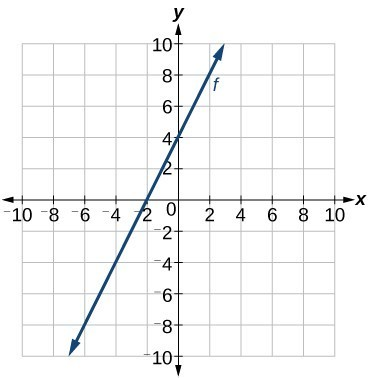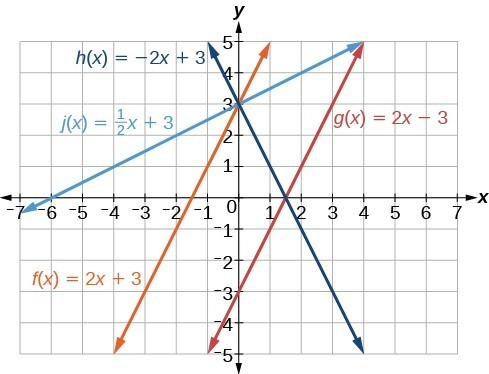## Write the equation for a linear function from the graph of a line

Recall that in Linear Functions, we wrote the equation for a linear function from a graph. Now we can extend what we know about graphing linear functions to analyze graphs a little more closely. Begin by taking a look at Figure 8. We can see right away that the graph crosses the y-axis at the point (0, 4) so this is the y-intercept.Figure 8

Then we can calculate the slope by finding the rise and run. We can choose any two points, but let’s look at the point (–2, 0). To get from this point to the y-intercept, we must move up 4 units (rise) and to the right 2 units (run). So the slope must be

$m=\frac{\text{rise}}{\text{run}}=\frac{4}{2}=2$

Substituting the slope and y-intercept into the slope-intercept form of a line gives

$y=2x+4$

### How To: Given a graph of linear function, find the equation to describe the function.

1. Identify the y-intercept of an equation.
2. Choose two points to determine the slope.
3. Substitute the y-intercept and slope into the slope-intercept form of a line.

### Example 4: Matching Linear Functions to Their Graphs

Match each equation of the linear functions with one of the lines in Figure 9.

1. $f\left(x\right)=2x+3$
2. $g\left(x\right)=2x - 3$
3. $h\left(x\right)=-2x+3$
4. $j\left(x\right)=\frac{1}{2}x+3$

### Solution

Analyze the information for each function.

1. This function has a slope of 2 and a y-intercept of 3. It must pass through the point (0, 3) and slant upward from left to right. We can use two points to find the slope, or we can compare it with the other functions listed. Function g has the same slope, but a different y-intercept. Lines I and III have the same slant because they have the same slope. Line III does not pass through (0, 3) so f must be represented by line I.
2. This function also has a slope of 2, but a y-intercept of –3. It must pass through the point (0, –3) and slant upward from left to right. It must be represented by line III.
3. This function has a slope of –2 and a y-intercept of 3. This is the only function listed with a negative slope, so it must be represented by line IV because it slants downward from left to right.
4. This function has a slope of $\frac{1}{2}$ and a y-intercept of 3. It must pass through the point (0, 3) and slant upward from left to right. Lines I and II pass through (0, 3), but the slope of j is less than the slope of f so the line for j must be flatter. This function is represented by Line II.

Now we can re-label the lines as in Figure 10.Figure 10